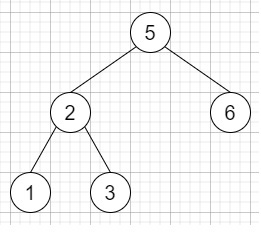# Verify Preorder Sequence in Binary Search Tree in C++

Suppose we have a sequence of numbers; we have to check whether it is the correct preorder traversal sequence of a binary search tree. We can assume that each number in the sequence is unique. Consider the following binary search tree −So, if the input is like [5,2,1,3,6], then the output will be true

To solve this, we will follow these steps −

• itr := -1

• low := -infinity

• for initialize i := 0, when i < size of preorder, update (increase i by 1), do −

• x := preorder[i]

• if x < low, then −

• return false

• while (itr >= 0 and preorder[itr] < x), do −

• low := preorder[itr]

• (decrease itr by 1)

• (increase itr by 1)

• preorder[itr] := x

• return true

## Example

Let us see the following implementation to get a better understanding −

Live Demo

#include <bits/stdc++.h>
using namespace std;
class Solution {
public:
bool verifyPreorder(vector<int<& preorder) {
int itr = -1;
int low = INT_MIN;
for (int i = 0; i < preorder.size(); i++) {
int x = preorder[i];
if (x < low)
return false;
while (itr >= 0 && preorder[itr] < x) {
low = preorder[itr];
itr--;
}
itr++;
preorder[itr] = x;
}
return true;
}
};
main(){
Solution ob;
vector<int< v = {5,2,1,3,6};
cout << (ob.verifyPreorder(v));
}

## Input

{5,2,1,3,6}

## Output

1